# RD Sharma Solutions for Class 6 Maths Chapter 2: Playing with Numbers Exercise 2.3

## RD Sharma Solutions for Class 6 Maths Exercise 2.3 PDF

RD Sharma Solutions are created with the aim of making the subject easy for students. The solutions are used by students while solving the exercise wise problems of RD Sharma textbook. Exercise 2.3 is the third chapter which provides the students with basic knowledge of prime and composite numbers. The faculty at BYJU’S creates solutions based on CBSE guidelines which help students in covering the entire syllabus. The answers are designed based on the understanding level of students. RD Sharma Solutions for Class 6 Maths Chapter 2 Playing with Numbers Exercise 2.3 are provided here.

## RD Sharma Solutions for Class 6 Maths Chapter 2: Playing with Numbers Exercise 2.3 Download PDF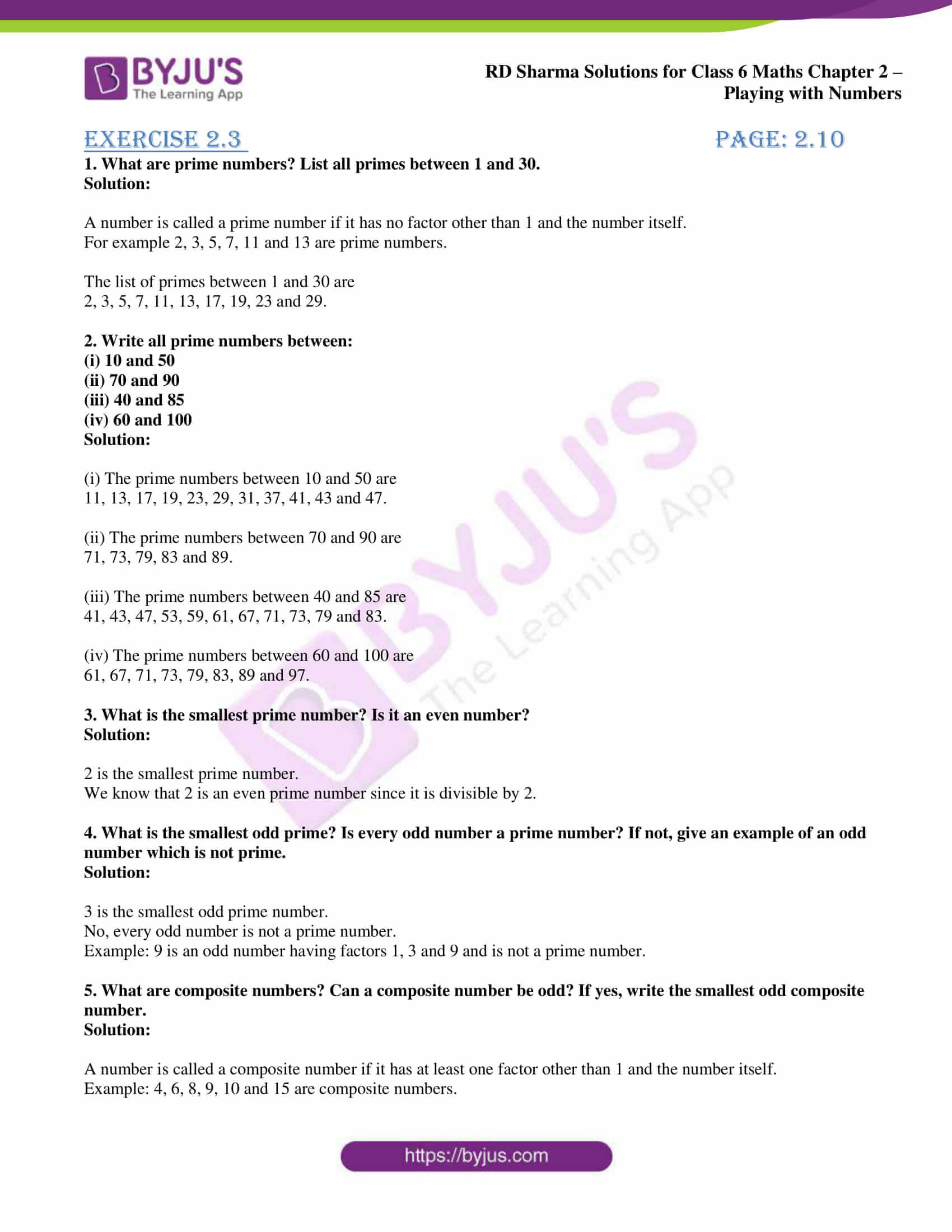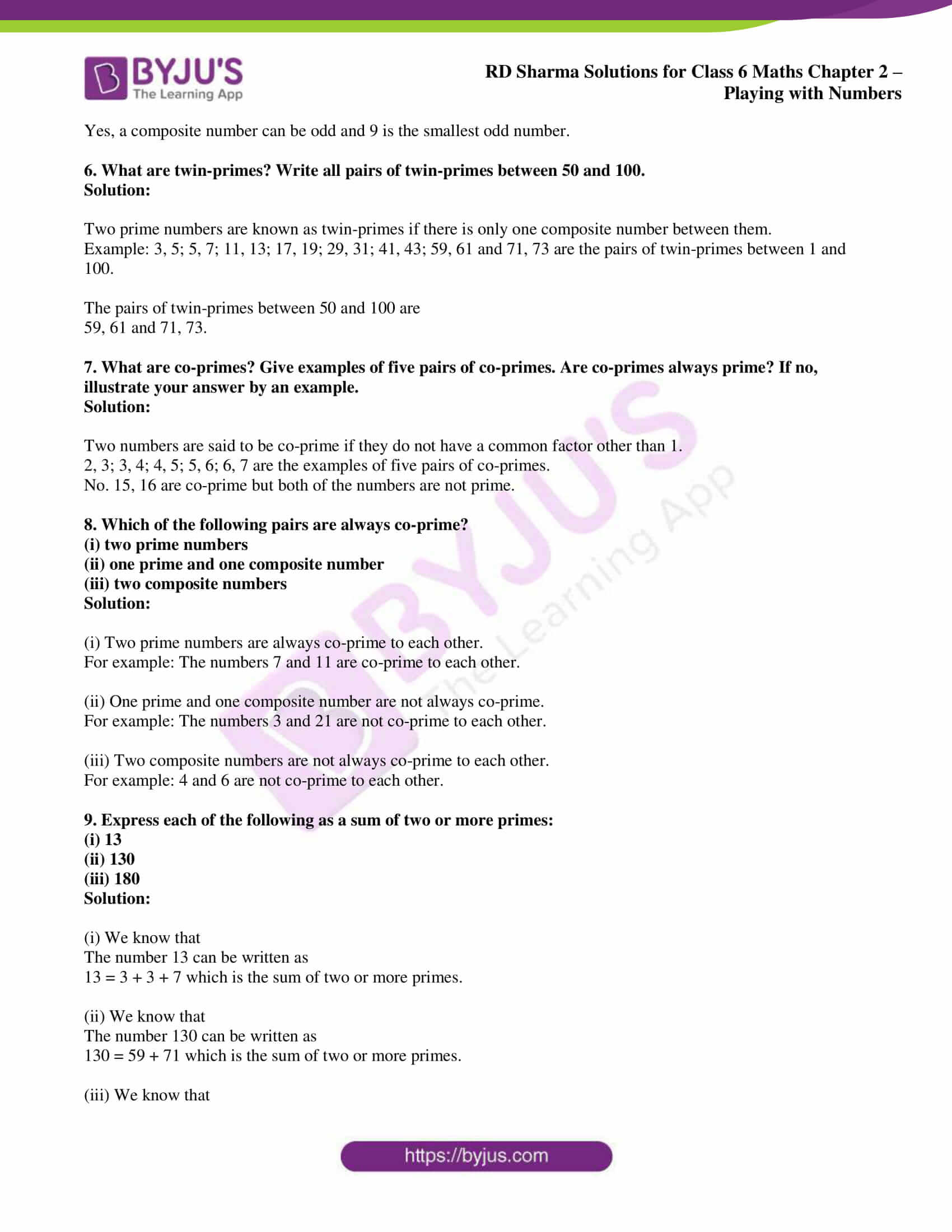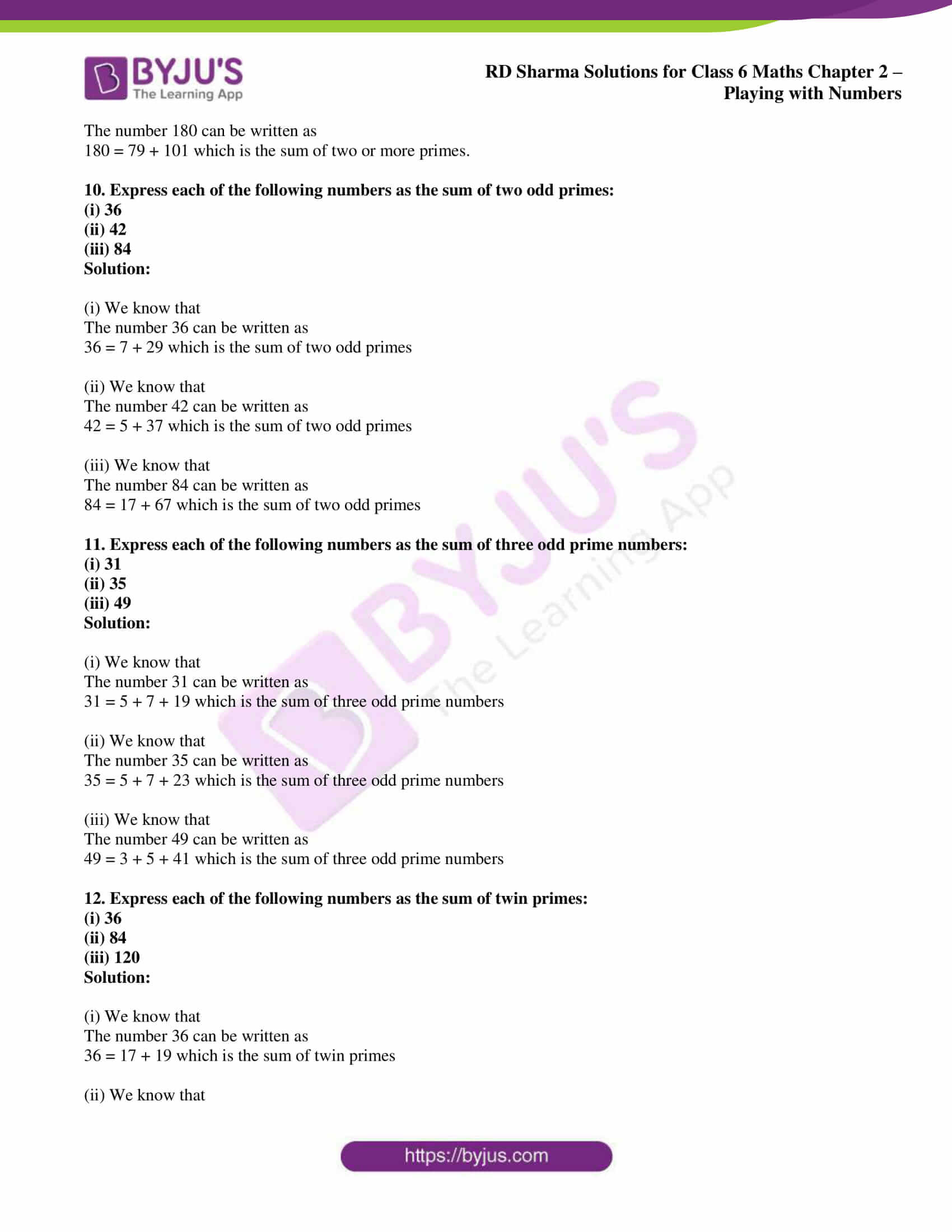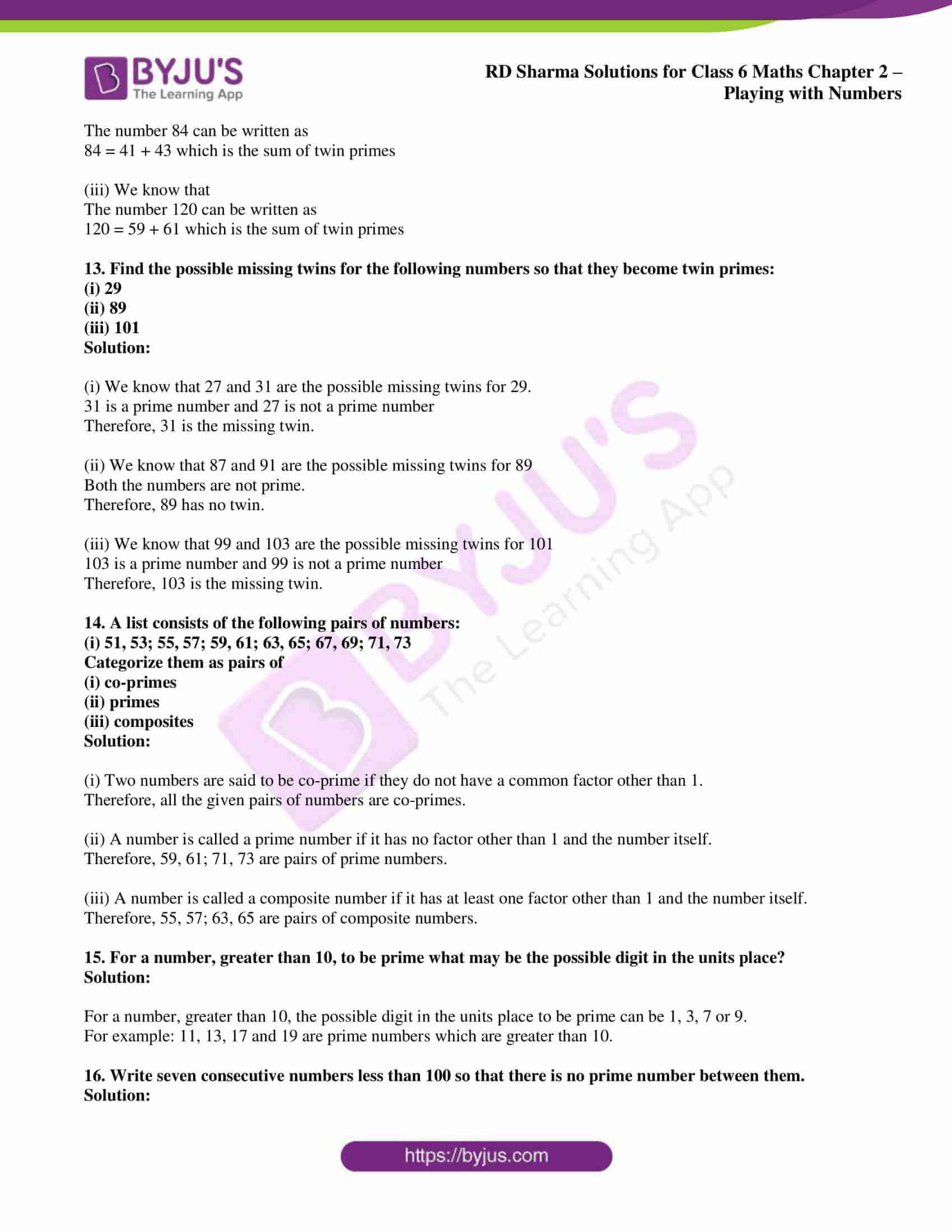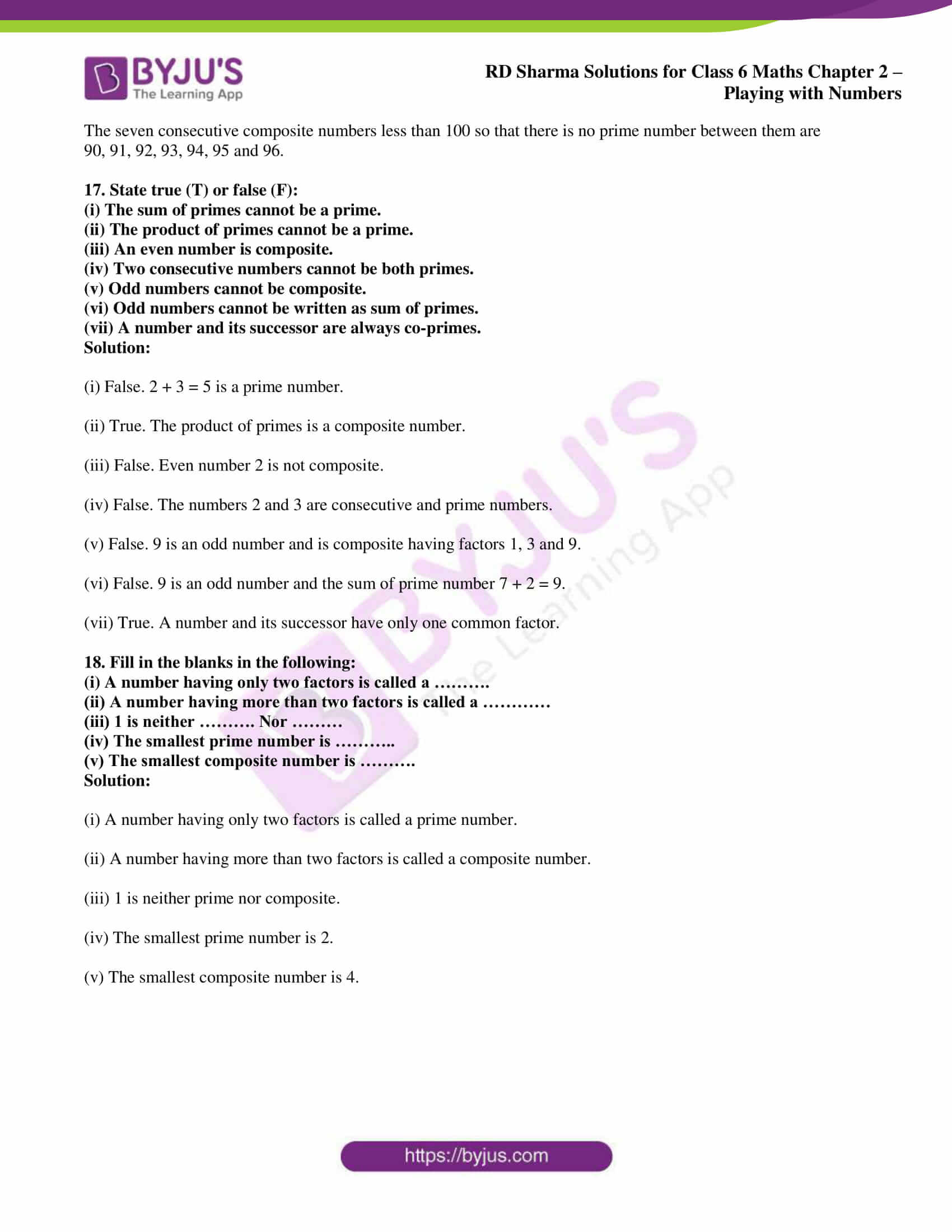## Access answers to Maths RD Sharma Solutions for Class 6 Chapter 2: Playing with Numbers Exercise 2.3

1. What are prime numbers? List all primes between 1 and 30.

Solution:

A number is called a prime number if it has no factor other than 1 and the number itself.

For example 2, 3, 5, 7, 11 and 13 are prime numbers.

The list of primes between 1 and 30 are

2, 3, 5, 7, 11, 13, 17, 19, 23 and 29.

2. Write all prime numbers between:

(i) 10 and 50

(ii) 70 and 90

(iii) 40 and 85

(iv) 60 and 100

Solution:

(i) The prime numbers between 10 and 50 are

11, 13, 17, 19, 23, 29, 31, 37, 41, 43 and 47.

(ii) The prime numbers between 70 and 90 are

71, 73, 79, 83 and 89.

(iii) The prime numbers between 40 and 85 are

41, 43, 47, 53, 59, 61, 67, 71, 73, 79 and 83.

(iv) The prime numbers between 60 and 100 are

61, 67, 71, 73, 79, 83, 89 and 97.

3. What is the smallest prime number? Is it an even number?

Solution:

2 is the smallest prime number.

We know that 2 is an even prime number since it is divisible by 2.

4. What is the smallest odd prime? Is every odd number a prime number? If not, give an example of an odd number which is not prime.

Solution:

3 is the smallest odd prime number.

No, every odd number is not a prime number.

Example: 9 is an odd number having factors 1, 3 and 9 and is not a prime number.

5. What are composite numbers? Can a composite number be odd? If yes, write the smallest odd composite number.

Solution:

A number is called a composite number if it has at least one factor other than 1 and the number itself.

Example: 4, 6, 8, 9, 10 and 15 are composite numbers.

Yes, a composite number can be odd and 9 is the smallest odd number.

6. What are twin-primes? Write all pairs of twin-primes between 50 and 100.

Solution:

Two prime numbers are known as twin-primes if there is only one composite number between them.

Example: 3, 5; 5, 7; 11, 13; 17, 19; 29, 31; 41, 43; 59, 61 and 71, 73 are the pairs of twin-primes between 1 and 100.

The pairs of twin-primes between 50 and 100 are

59, 61 and 71, 73.

7. What are co-primes? Give examples of five pairs of co-primes. Are co-primes always prime? If no, illustrate your answer by an example.

Solution:

Two numbers are said to be co-prime if they do not have a common factor other than 1.

2, 3; 3, 4; 4, 5; 5, 6; 6, 7 are the examples of five pairs of co-primes.

No. 15, 16 are co-prime but both of the numbers are not prime.

8. Which of the following pairs are always co-prime?

(i) two prime numbers

(ii) one prime and one composite number

(iii) two composite numbers

Solution:

(i) Two prime numbers are always co-prime to each other.

For example: The numbers 7 and 11 are co-prime to each other.

(ii) One prime and one composite number are not always co-prime.

For example: The numbers 3 and 21 are not co-prime to each other.

(iii) Two composite numbers are not always co-prime to each other.

For example: 4 and 6 are not co-prime to each other.

9. Express each of the following as a sum of two or more primes:

(i) 13

(ii) 130

(iii) 180

Solution:

(i) We know that

The number 13 can be written as

13 = 3 + 3 + 7 which is the sum of two or more primes.

(ii) We know that

The number 130 can be written as

130 = 59 + 71 which is the sum of two or more primes.

(iii) We know that

The number 180 can be written as

180 = 79 + 101 which is the sum of two or more primes.

10. Express each of the following numbers as the sum of two odd primes:

(i) 36

(ii) 42

(iii) 84

Solution:

(i) We know that

The number 36 can be written as

36 = 7 + 29 which is the sum of two odd primes

(ii) We know that

The number 42 can be written as

42 = 5 + 37 which is the sum of two odd primes

(iii) We know that

The number 84 can be written as

84 = 17 + 67 which is the sum of two odd primes

11. Express each of the following numbers as the sum of three odd prime numbers:

(i) 31

(ii) 35

(iii) 49

Solution:

(i) We know that

The number 31 can be written as

31 = 5 + 7 + 19 which is the sum of three odd prime numbers

(ii) We know that

The number 35 can be written as

35 = 5 + 7 + 23 which is the sum of three odd prime numbers

(iii) We know that

The number 49 can be written as

49 = 3 + 5 + 41 which is the sum of three odd prime numbers

12. Express each of the following numbers as the sum of twin primes:

(i) 36

(ii) 84

(iii) 120

Solution:

(i) We know that

The number 36 can be written as

36 = 17 + 19 which is the sum of twin primes

(ii) We know that

The number 84 can be written as

84 = 41 + 43 which is the sum of twin primes

(iii) We know that

The number 120 can be written as

120 = 59 + 61 which is the sum of twin primes

13. Find the possible missing twins for the following numbers so that they become twin primes:

(i) 29

(ii) 89

(iii) 101

Solution:

(i) We know that 27 and 31 are the possible missing twins for 29.

31 is a prime number and 27 is not a prime number

Therefore, 31 is the missing twin.

(ii) We know that 87 and 91 are the possible missing twins for 89

Both the numbers are not prime.

Therefore, 89 has no twin.

(iii) We know that 99 and 103 are the possible missing twins for 101

103 is a prime number and 99 is not a prime number

Therefore, 103 is the missing twin.

14. A list consists of the following pairs of numbers:

(i) 51, 53; 55, 57; 59, 61; 63, 65; 67, 69; 71, 73

Categorize them as pairs of

(i) co-primes

(ii) primes

(iii) composites

Solution:

(i) Two numbers are said to be co-prime if they do not have a common factor other than 1.

Therefore, all the given pairs of numbers are co-primes.

(ii) A number is called a prime number if it has no factor other than 1 and the number itself.

Therefore, 59, 61; 71, 73 are pairs of prime numbers.

(iii) A number is called a composite number if it has at least one factor other than 1 and the number itself.

Therefore, 55, 57; 63, 65 are pairs of composite numbers.

15. For a number, greater than 10, to be prime what may be the possible digit in the units place?

Solution:

For a number, greater than 10, the possible digit in the units place to be prime can be 1, 3, 7 or 9.

For example: 11, 13, 17 and 19 are prime numbers which are greater than 10.

16. Write seven consecutive numbers less than 100 so that there is no prime number between them.

Solution:

The seven consecutive composite numbers less than 100 so that there is no prime number between them are

90, 91, 92, 93, 94, 95 and 96.

17. State true (T) or false (F):

(i) The sum of primes cannot be a prime.

(ii) The product of primes cannot be a prime.

(iii) An even number is composite.

(iv) Two consecutive numbers cannot be both primes.

(v) Odd numbers cannot be composite.

(vi) Odd numbers cannot be written as sum of primes.

(vii) A number and its successor are always co-primes.

Solution:

(i) False. 2 + 3 = 5 is a prime number.

(ii) True. The product of primes is a composite number.

(iii) False. Even number 2 is not composite.

(iv) False. The numbers 2 and 3 are consecutive and prime numbers.

(v) False. 9 is an odd number and is composite having factors 1, 3 and 9.

(vi) False. 9 is an odd number and the sum of prime number 7 + 2 = 9.

(vii) True. A number and its successor have only one common factor.

18. Fill in the blanks in the following:

(i) A number having only two factors is called a ……….

(ii) A number having more than two factors is called a …………

(iii) 1 is neither ………. Nor ………

(iv) The smallest prime number is ………..

(v) The smallest composite number is ……….

Solution:

(i) A number having only two factors is called a prime number.

(ii) A number having more than two factors is called a composite number.

(iii) 1 is neither prime nor composite.

(iv) The smallest prime number is 2.

(v) The smallest composite number is 4.

### RD Sharma Solutions for Class 6 Maths Chapter 2 – Playing with Numbers Exercise 2.3

RD Sharma Solutions Class 6 Maths Chapter 2 Playing with Numbers Exercise 2.3 helps us understand the ways of finding prime and composite numbers along with methods used while answering the questions.

### Key features of RD Sharma Solutions for Class 6 Maths Chapter 2: Playing with Numbers Exercise 2.3

• RD Sharma Solutions are created by the subject matter experts at BYJU’S after conducting wide research on each concept.
• The PDF can be downloaded by students and used as a vital resource to boost up their exam preparation.
• The solutions mainly improve conceptual knowledge among students, which is important to solve problems.
• The faculty at BYJU’S design the solutions based on the latest CBSE guidelines and exam pattern.